Courses

# Test: Graphs Algorithms- 1

## 15 Questions MCQ Test RRB JE for Computer Science Engineering | Test: Graphs Algorithms- 1

Description
This mock test of Test: Graphs Algorithms- 1 for Computer Science Engineering (CSE) helps you for every Computer Science Engineering (CSE) entrance exam. This contains 15 Multiple Choice Questions for Computer Science Engineering (CSE) Test: Graphs Algorithms- 1 (mcq) to study with solutions a complete question bank. The solved questions answers in this Test: Graphs Algorithms- 1 quiz give you a good mix of easy questions and tough questions. Computer Science Engineering (CSE) students definitely take this Test: Graphs Algorithms- 1 exercise for a better result in the exam. You can find other Test: Graphs Algorithms- 1 extra questions, long questions & short questions for Computer Science Engineering (CSE) on EduRev as well by searching above.
QUESTION: 1

### Consider the directed graph shown in the figure below. There are multiple shortest paths between vertices  and . Which one will be reported by Dijkstra’s shortest path algorithm? Assume that, in any iteration, the shortest path to a vertex  is updated only when a strictly shorter path to  is discovered.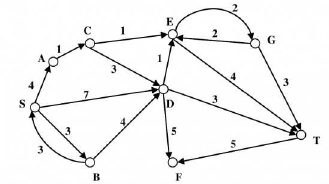Solution:

Relaxation at every vertex is as follows
Note the next vertex is taken out here is in RED colour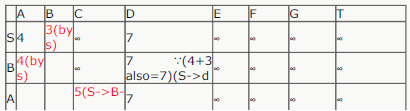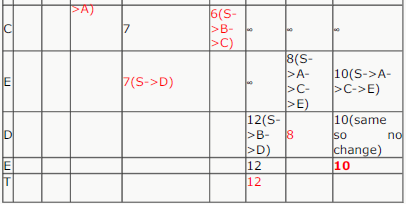Now We see for S to T its (S->A->C->E->.T)
which is Option d

QUESTION: 2

Solution:
QUESTION: 3

### What is the time complexity of Bellman-Ford single-source shortest path algorithm on a complete graph of n vertices?

Solution:

Time complexity of Bellman-Ford algorithm is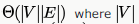is number of vertices and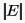is number of edges. If the graph is complete, the value ofbecomes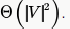So overall time complexity becomes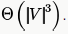And given here is n vertices. So answers ends up to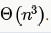QUESTION: 4

Consider the directed graph below given.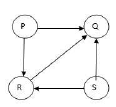Which one of the following is TRUE?

Solution:

The C option has been copied wrongly
C) Both PSRQ and SPRQ are topological orderings
i)Apply DFS by choosing P or S as starting vertices
ii)As the vertex gets a finishing time assign it to the head of a linked list

QUESTION: 5

Consider the tree arcs of a BFS traversal from a source node W in an unweighted, connected, undirected graph. The tree T formed by the tree arcs is a data structure for computing

Solution:

BFS always having starting node. It does not calculate shortest path between every pair but it computes shorted path between W and any other vertex ..

QUESTION: 6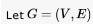be a simple undirected graph, and  be a particular vertex in it called the source.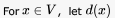denote the shortest distance in G from s to x. A breadth first search (BFS) is performed starting at s. Let T be the resultant BFS tree.  If (u,v) is an edge of G that is not in Tthen which one of the following CANNOT be the value of ,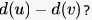Solution: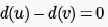is possible when both u and v have an edge from t and t is in the shortest path from s to u or v .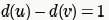is possible when v and t are in the shortest path from s to u and both t  and v are siblings- same distance from s to both t and v causing t-u to both and causing v-u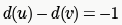is possible as explained above by interchanging u and v.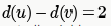is not possible. This is because on BFS traversal we either visit u first or v . Let's u take first. Now, we put all neighbors of u on queue. Since v is a neighbour and v is not visited before as assumed, d(v) will become d(u)+1

Similarly, for v being visited first.

QUESTION: 7

In an adjacency list representation of an undirected simple graph G = (V,E) , each edge (u,v)  has two adjacency list entries: [v] in the adjacency list of u , and [u] in the adjacency list of v. These are called twins of each other. A twin pointer is a pointer from an adjacency list entry to its twin. If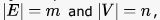, and the memory size is not a constraint, what is the time complexity of the most efficient algorithm to set the twin pointer in each entry in each adjacency list?

Solution:

Applying BFS on Undirected graph give you twin pointer .Visit every vertex level-wise for every vertex fill adjacent vertex in the adjacency list. BFS take 0(m+n) time.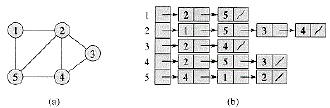take extra field for storing no. of linked list for perticular vertex. take extra m+n time( m vertex and n edges).

QUESTION: 8

Let G = (V,E) be  connected undirected edge-weighted graph. The weights of the edges in E are positive and distinct. Consider the following statements:

(I) Minimum Spanning Tree of G is always unique.

(II) Shortest path between any two vertices of G is always unique.

Which of the above statements is/are necessarily true?

Solution:

> MST is not unique only when edges are not distinct. Here the edges are distinct. Be careful for the keyword
DISTINCT.

> Shortest Path can be different even if the edges are distinct. Example is shown below. Shortest path from A
to C is not unique here.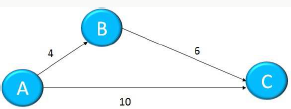QUESTION: 9

The Breadth First Search (BFS) algorithm has been implemented using the queue data structure. Which one of the following is a possible order of visiting the nodes in the graph below?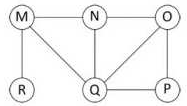Solution:

In BFS starting from a node, we traverse all node adjacent to it at first then repeat same for next nodes

Here you can see only D is following BFS sequence properly

As per BFS if we start from M then RQN has to come after it in any order but in A here O comes so it is not
As per BFS if we start from Nthen QMO has to come after it in any order but in A here P comes so it is not
As per BFS if we start from M then MNOP has to come after it in any order but in A here R comes so it is not but D following the sequences

QUESTION: 10

Let G be an undirected graph. Consider a depth-first traversal of G, and let T be the resulting depth-first search tree. Let u be a vertex in G and let v be the first new (unvisited) vertex visited after visiting u in the traversal. Which of the following statement is always true?

Solution: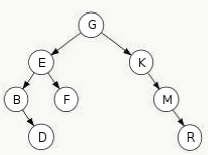let this be the dfs order of tree then
u= D and v = F
so what we conclude
1. its not necessary their is edge b/w them .
2. if thier is no edge then u must be leaf i.e. D is leaf here.
3. it not always possible u and v have same parent . but they have same ancestor .

QUESTION: 11

Consider the following directed graph.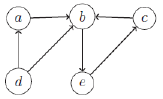Suppose a depth-first traversal of this graph is performed, assuming that whenever there is a choice, the vertex earlier in the alphabetical order is to be chosen. Suppose the number of tree edges is , the number of back edges is  and the number of cross edges is . Then

Solution:

Since they said that whenever their is a choice we will have to select the node which is alphabetically earlier , therefore we choose the starting node as A .

The tree then becomes A-B-E-C . Therefore no of tree edges is 3 that is (T=3) .
Now there is one cycle B-E-C so we will get a back edge from C to B while performing DFS. Hence B=1 .
Now D becomes disconnected node and it can only contribute in forming cross edge . There are 2 cross edges D-A , D-B .
Therefore C=2 .

QUESTION: 12

The minimum number of record movements required to merge five files A (with 10 records), B (with 20 records), C (with 15 records), D (with 5 records) and E (with 25 records) is:

Solution:

Arrange files in increasing order of records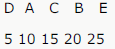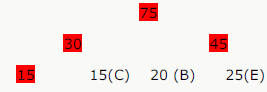5(D) 10(A)
No of movements=15+30+45+75=165

QUESTION: 13

We are given 9 tasks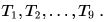The execution of each task requires one unit of time. We can execute one task at a time. Each task  Thas a profit Pi and a deadline di . Profit  Pis earned if the task is completed before the end of the dith unit of time.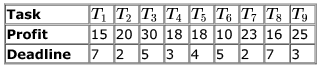Are all tasks completed in the schedule that gives maximum profit?

Solution:

The most important statement in question is

each task requires one unit of time

This shows that we can greedily choose the better task and that should give us the optimal solution. The best task would be the one with maximum profit. Thus we can sort the tasks based on deadline and then profit as follows: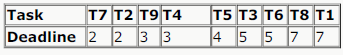0 ----T7 ----- 1----T2-----2-----T9-----3-----T5-----4-----T3-----5-----T8-----6-----T1------7

T4 and T6 left out

QUESTION: 14

We are given 9 tasksThe execution of each task requires one unit of time. We can execute one task at a time. Each task  Thas a profit Pi and a deadline di . Profit  Pis earned if the task is completed before the end of the dith unit of time.What is the maximum profit earned?

Solution:

The most important statement in question is

each task requires one unit of time

This shows that we can greedily choose the better task and that should give us the optimal solution. The best task would be the one with maximum profit. Thus we can sort the tasks based on deadline and then profit as follows: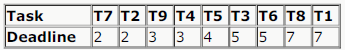0 --T7 -- 1 -- T2 -- 2 -- T9 -- 3 -- T5 -- 4 -- T3 -- 5 -- T8 -- 6 -- T1 -- 7

so we know that T4 and T6 are left
so profit will not include T4 and T6 = 15 + 20 +30 +18 + 16 + 23 + 25 = 147

QUESTION: 15

For which of the following combinations of the degrees of vertices would the connected graph be eulerian?

Solution:

A graph is eulerian if either all of its vertices are even or if only two of its vertices are odd.